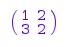#### Linear Algebra in MATLAB

Linear algebra:

Linear algebra is the branch of mathematics. It is about vector spaces, often finite or countable infinite dimensional, plus linear mappings among such spaces. Linear algebra is essential to both applied and pure mathematics. For example, abstract algebra occurs by relaxing the axioms of a vector space. Linear equations are logically represented by using the formalism of vectors and matrices.

The main objective of this topic is to become people familiar with the concept of using a MATLAB to perform linear algebra. MATLAB is a program which performs linear algebra very well, and it is very frequently used by mathematicians, engineers and scientists etc. It helps to solve problems that could be impractical to answer by hand.

We can number of operations in linear algebra like analysis of matrix, linear equations, singular values, logarithms, factorization, exponentials etc. The MATLAB library contains more than 40 functions for linear algebra such as info(linalg).

Library 'linalg': the linear algebra package:

-- Interface:

linalg::basis,            linalg::charmat,

After exported, library functions could be used by their short notation. The function call use(linalg) exports all functions of linalg. After exporting this function one can use the the other functions by their short form like name gaussElim can be used instead of linalg::gaussElim.

The simple way to describe a matrix A is using the command matrix. The syntax of defining a 2 ? 2 matrix is as follows:

A := matrix ([[1, 2], [3, 2]])Now after defining matrices we can perform different operations like multiplication, inverse, Exponential of matrices, adjoint and transpose of matrix etc.

Various processes from linear algebra are as well used in analytic geometry, engineering, physics, computer science, computer animation, and in economics. Because linear algebra is such a well-managed theory, nonlinear mathematical models are occasionally estimated by linear ones. We can plot complex functions by plotting commands with functions of a complex variable. By using MATLAB we can define different complex numbers in linear algebra like, Cartesian form, polar form, arithmetic with complex form, complex conjugate, real and imaginary, modulus and angle etc.

Latest technology based Matlab Programming Online Tutoring Assistance

Tutors, at the www.tutorsglobe.com, take pledge to provide full satisfaction and assurance in Matlab Programming help via online tutoring. Students are getting 100% satisfaction by online tutors across the globe. Here you can get homework help for Matlab Programming, project ideas and tutorials. We provide email based Matlab Programming help. You can join us to ask queries 24x7 with live, experienced and qualified online tutors specialized in Matlab Programming. Through Online Tutoring, you would be able to complete your homework or assignments at your home. Tutors at the TutorsGlobe are committed to provide the best quality online tutoring assistance for Matlab Programming Homework help and assignment help services. They use their experience, as they have solved thousands of the Matlab Programming assignments, which may help you to solve your complex issues of Matlab Programming. TutorsGlobe assure for the best quality compliance to your homework. Compromise with quality is not in our dictionary. If we feel that we are not able to provide the homework help as per the deadline or given instruction by the student, we refund the money of the student without any delay.